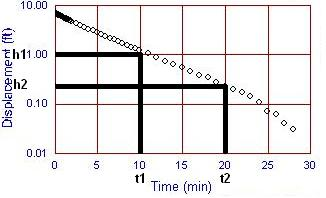## Calculator 11 - Slugtest Calculator

###BOUWER/RICE SLUGTEST CALCULATOR  Calculate Hydraulic Conductivity from slugtest data using Bouwer and Rice method STEP1 - Graph slugtest data - Log Head Vs. Linear Time.  Read h1,h2,t1,t2 from straight line part of curve.STEP2 - Look up well construction paramters.STEP3 - Enter test parameters. h1 (head at time t=t1):  h2 (head at time t=t2):  t1 (time at head h1):  t2 (time at head h2):  Well Type:   fully penetrating well partially penetrating well b (Aquifer saturated thickness):   H (dist. from w. t. to bottom of pack): L (length of the screened interval): rc (radius of casing): rw (radius of well): Hydraulic Conductivity:     Example CalculationSTEP1 - Graph slugtest data - Log Head Vs. Linear Time.STEP 2 & 3 - Enter test parameters. h1 = 1.0 ft h2 = 0.2 ft t1 = 10 min t2  = 20 min Well Type:  = Partially Penetrating b = 100 ft H = 50 ft L = 15 ft rc = 0.083 ft rw = 0.33 ft Hydraulic Conductivity =   0.00011 ft/min

Single Well Solutions

If you are looking for a less expensive Win 98/XP software try